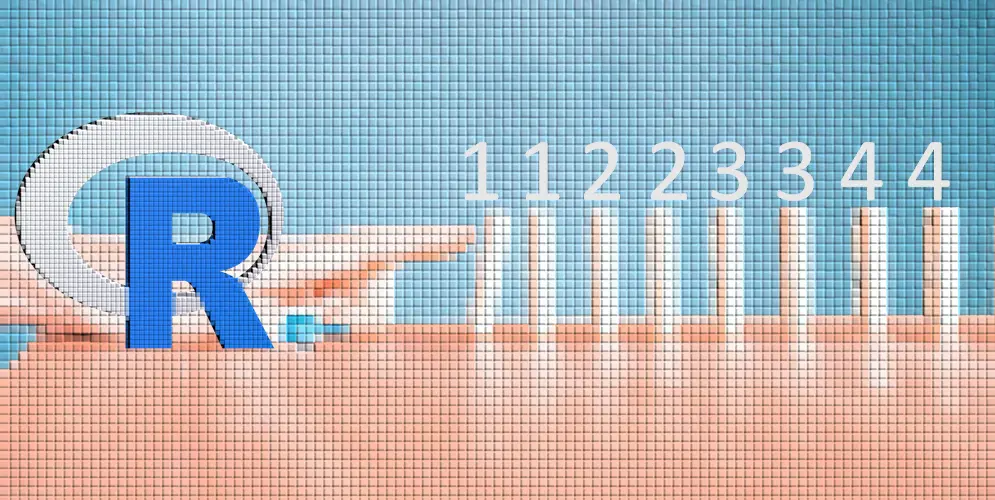# How to create a repeated number sequence in R

Sometimes it is necessary to generate not only a simple number sequence but a repeated number sequence in R. Here is how to do that.

The function which can generate sequences in R is seq. In a simple situation, you can use other base R capabilities. For example, if I want to generate numbers from 1 to 10, it looks like this.

```1:10

#  1  2  3  4  5  6  7  8  9 10```

When it is a more complicated scenario, and it is necessary to use specific steps in the sequence, try to use function seq. For example, if I want to generate numbers from 1 to 10 using a specific step, it looks like this.

```seq(from = 1, to = 10, by = 1.5)

#  1.0  2.5  4.0  5.5  7.0  8.5 10.0```

By using this function, you can generate sequences not only with numbers but also with dates and time intervals in R.

## Repeated number sequence in R

R base function rep is capable of generating replications in R. To repeat numbers in a sequence, you can use the function rep and specify argument each. Here is a simple example of creating a sequence from 1 to 5.

```rep(1:5, each = 2)

# 1 1 2 2 3 3 4 4 5 5```

If you add to that function seq, you can get a repeated number sequence in R with a specific step within that.

```rep(seq(from = 1, to = 10, by = 1.5), each = 2)

#  1.0  1.0  2.5  2.5  4.0  4.0  5.5  5.5  7.0  7.0  8.5  8.5 10.0 10.0```

There are also argument times in the rep function. By using that, you can repeat the whole sequence, and the result looks different from the previous one.

```rep(1:5, times = 2)

# 1 1 2 2 3 3 4 4 5 5```

In other scenarios, you might be interested in how to create a column with an index in R.

Categories R